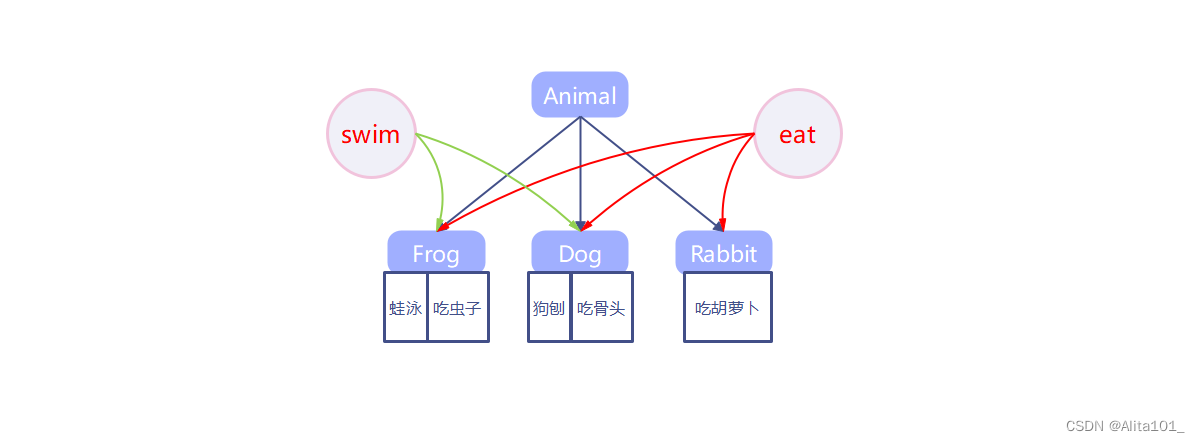# JAVA随机数之多种方法从给定范围内随机N个不重复数

+关注继续查看

1、在j2se中使用Math.random()令系统随机选取一个0~1之间的double类型小数，将其乘以一个数，比如25，就能得到一个0~25范围内的随机数，这个在j2me中没有；

Java代码
1. int randomNumber = (int) Math.round(Math.random()*(max-min)+min);

2、在System类中有一个currentTimeMillis()方法，这个方法返回从1970年1月1号0点0分0秒到目前的一个long型的毫秒 数，可作为一个随机数，还可以将其对某些数取模，就能限制随机数的范围；此方式在循环中同时产生多个随机数时，会是相同的值，有一定的局限性！

Java代码
1. long randomNum = System.currentTimeMillis();
2. int randomNumber = (int) randomNum%(max-min)+min;

3、使用java.util.Random类来产生一个随机数发生器，这个也是我们在j2me的程序里经常用的一个取随机数的方法。它有两种形式的构造函 数，分别是Random()和Random(long seed)。Random()使用当前时间即System.currentTimeMillis()作为发生器的种子，Random(long seed)使用指定的seed作为发生器的种子。随机数发生器(Random)对象产生以后，通过调用不同的method：nextInt()、 nextLong()、nextFloat()、nextDouble()等获得不同类型随机数。 如果两个Random对象使用相同的种子（比如都是25），并且以相同的顺序调用相同的函数，那它们返回值完全相同。

Java代码
1. Random random = new Random();
2. int randomNumber =  random.nextInt(max)%(max-min+1) + min;

1、方法一：最简单最易理解的两重循环去重

Java代码
1. /**
2.  * 随机指定范围内N个不重复的数
3.  * 最简单最基本的方法
4.  * @param min 指定范围最小值
5.  * @param max 指定范围最大值
6.  * @param n 随机数个数
7.  */
8. public static int[] randomCommon(int min, int max, int n){
9.     if (n > (max - min + 1) || max < min) {
10.            return null;
11.        }
12.     int[] result = new int[n];
13.     int count = 0;
14.     while(count < n) {
15.         int num = (int) (Math.random() * (max - min)) + min;
16.         boolean flag = true;
17.         for (int j = 0; j < n; j++) {
18.             if(num == result[j]){
19.                 flag = false;
20.                 break;
21.             }
22.         }
23.         if(flag){
24.             result[count] = num;
25.             count++;
26.         }
27.     }
28.     return result;
29. }

2、方法二：利用HashSet的特征，只能存放不同的值

Java代码
1. /**
2.  * 随机指定范围内N个不重复的数
3.  * 利用HashSet的特征，只能存放不同的值
4.  * @param min 指定范围最小值
5.  * @param max 指定范围最大值
6.  * @param n 随机数个数
7.  * @param HashSet<Integer> set 随机数结果集
8.  */
9.    public static void randomSet(int min, int max, int n, HashSet<Integer> set) {
10.        if (n > (max - min + 1) || max < min) {
11.            return;
12.        }
13.        for (int i = 0; i < n; i++) {
14.            // 调用Math.random()方法
15.            int num = (int) (Math.random() * (max - min)) + min;
17.        }
18.        int setSize = set.size();
19.        // 如果存入的数小于指定生成的个数，则调用递归再生成剩余个数的随机数，如此循环，直到达到指定大小
20.        if (setSize < n) {
21.         randomSet(min, max, n - setSize, set);// 递归
22.        }
23.    }

3、方法三：排除已随机到的数

Java代码
1. /**
2.  * 随机指定范围内N个不重复的数
3.  * 在初始化的无重复待选数组中随机产生一个数放入结果中，
4.  * 将待选数组被随机到的数，用待选数组(len-1)下标对应的数替换
5.  * 然后从len-2里随机产生下一个随机数，如此类推
6.  * @param max  指定范围最大值
7.  * @param min  指定范围最小值
8.  * @param n  随机数个数
9.  * @return int[] 随机数结果集
10.  */
11. public static int[] randomArray(int min,int max,int n){
12.     int len = max-min+1;
13.
14.     if(max < min || n > len){
15.         return null;
16.     }
17.
18.     //初始化给定范围的待选数组
19.     int[] source = new int[len];
20.        for (int i = min; i < min+len; i++){
21.         source[i-min] = i;
22.        }
23.
24.        int[] result = new int[n];
25.        Random rd = new Random();
26.        int index = 0;
27.        for (int i = 0; i < result.length; i++) {
28.         //待选数组0到(len-2)随机一个下标
29.            index = Math.abs(rd.nextInt() % len--);
30.            //将随机到的数放入结果集
31.            result[i] = source[index];
32.            //将待选数组中被随机到的数，用待选数组(len-1)下标对应的数替换
33.            source[index] = source[len];
34.        }
35.        return result;
36. }

调用实例：

Java代码
1.     public static void main(String[] args) {
2.     int[] reult1 = randomCommon(20,50,10);
3.     for (int i : reult1) {
4.         System.out.println(i);
5.     }
6.
7.     int[] reult2 = randomArray(20,50,10);
8.     for (int i : reult2) {
9.         System.out.println(i);
10.     }
11.
12.     HashSet<Integer> set = new HashSet<Integer>();
13.     randomSet(20,50,10,set);
14.        for (int j : set) {
15.         System.out.println(j);
16.     }
17. }Java String 的 常量池 和 intern 方法 简析
Java String 的 常量池 和 intern 方法 简析字符串常量池和 intern 方法 先举个例子，我们创建 10000 个相同的 String，并且不使用常量池 String[] list = new String; for (int i = 0; i < 10000; i++) { list[i] = new String(new char[]{'a','b','c'}); } 结果是：每个 String 都用新开的对象，占用大量内存 现在我们这样创建，增加一个 map ，key 和 value 内容一样，每次取的时候，先检查一下 map 里面有没
45 038 0Java形参个数可变的方法

24 0Java的学习笔记（04）方法
Java方法01 方法是语句的集合，执行一个功能。方法再程序中被创建，再其他地方被引用 一个方法只做一个功能 方法的命名规则：首字母小写，驼峰规则。 package method; public class Demo01 { //mian 方法 public static void main(String[] args) { int sum = add(1,2); //调用一个方法：加法 // 实际参数：实际调用传递给
17 0【Java编程进阶】方法初识

35 023 0java二分查找方法的实现和其优化（解决整数溢出）
java二分查找方法的实现和其优化（解决整数溢出）
36 0Java练习题——抽象类、方法以及接口

31 0Java练习题——方法（基础版）

90 0

Java高级开发31518156

Java零基础入门22326

Java Web开发系列课程 - Struts2框架入门49249

Java Web开发系列课程 - Spring框架入门2976925

Java Web开发-Web应用、Tomcat、HTTP请求与响应137117

Java Spring Boot 2.6.0开发实战-1024程序员节创造营公益课9315

Java工程师必读手册

Java应用提速（速度与激情）

Java单元测试实战